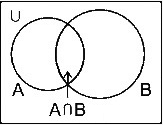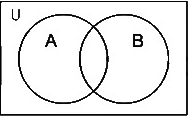If P and Q be two sets such that P ∪ Q = P, ...

### Related TestIf P and Q be two sets such that P ∪ Q = P, then P ∩ Q will be:Kunaal SatijaIntersection
Let A and B be two sets. The intersection of A and B is the set of all those elements which are present in both sets A and B.
The intersection of A and B is denoted by A ∩ B
i.e., A ∩ B = {x : x ∈ A and x ∈ B}
The Venn diagram for intersection is as shown below:Union:
Let A and B be two sets. The union of A and B is the set of all those elements which belong to either A or B or both A and B.
The union of A and B is denoted by A ∪ B.
i.e., A ∪ B = {x : x ∈ A or x ∈ B}
The Venn diagram for the union of any two sets is shown below:A ∪ B = A + B - A ∩ B
As we know,
P ∪ Q = P + Q - P ∩ Q
Putting the values given in the question,
P = P + Q - P ∩ Q
P ∩ Q = Q
Hence, the correct answer is Q.View courses related to this question Explore CAT courses
 Explore CAT coursesView courses related to this question1 Crore+ students have signed up on EduRev. Have you?

• ### Directions [Set of 5 questions]: Read the following passage carefully and a... more(Scan QR code)